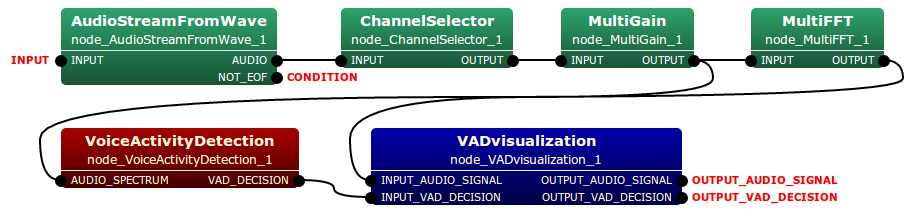# VoiceActivityDetection Node¶

## Outline of the node¶

This node delimits the a speech-present period.

## Typical connection¶

This node is connected with the VoiceActivityDetection node. Typical connection of this node is depicted as follows:## Input-output and property of the node¶

### Input¶

AUDIO_SPECTRUM Matrixd<complex<float> >
Windowed spectrum data. A row index is channel, and a column index is frequency.

### Output¶

Decision of speech-present frame

### Parameters¶

Parameters of this node are listed as follows:

Parameter name Type Default value Unit Description
VAD_NOISE_DURATION float 3.0 second Time duration to be regarded as “noise” from the first frame
VAD_THRESHOLD float 50.0   Threshold for voice activity decision.
ADVANCE int 160 sample The length in sample between a frame and a previous frame.
SAMPLING_RATE int 16000 Hz Sampling rate.

## Detail of the node¶

This node estimates the voice activity by using log likelifood ratio of speech and noise variances of the zero-mean Gaussian statistical model . Let $$X_{l/r}\left[f, n\right]$$ be an input audio signal at frequency bin $$f$$ and time frame $$n$$, this method regards speech-present when following equation is satisfied:

$$\frac{1}{F} \sum^{F}_{f=1} \gamma \left[f,n\right]- {\rm log} \gamma \left[f,n\right] -1 > \eta_{VAD}$$,

$$\lambda_N \left[f\right] = E\left|N_l\left[f\right] \cdot N_r\left[f\right]^{\ast}\right|$$,

$$\gamma\left[f,n\right]=\left|X_l \left[f,n\right] \cdot X_r\left[f,n\right]^{\ast}\right|/ \lambda_N\left[f\right]$$,

where $$N\left[f\right]$$ and $$\eta_{VAD}$$ represent the variance of a estimated noise and threshold parameter, respectively.